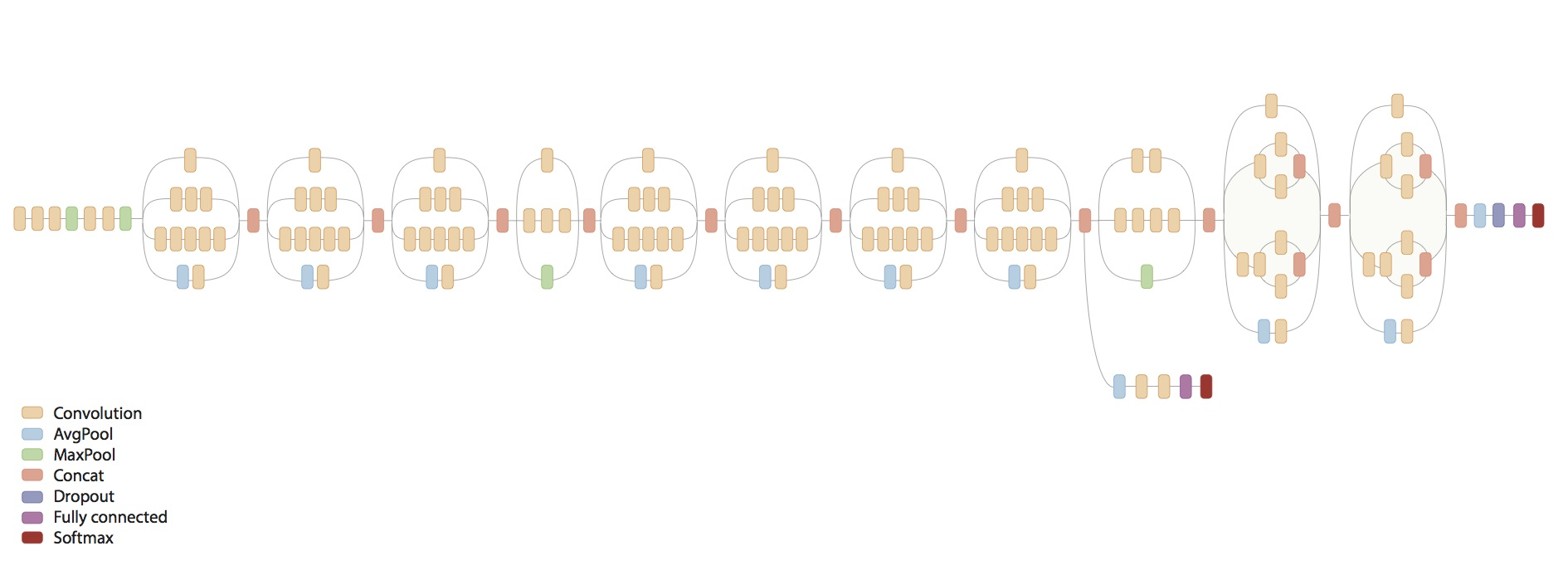## CNN Models

How to

The LeNet model is one of the most simple model architecture. We start with an input that is two dimension since the LeNet is trained on black and white inputs. All convolution layers in the LeNet uses a 5x5 convolution without padding since padding. For pooling layers, the model uses an average pooling. After the last pooling layer, it is then attached with a fully connected layer and finally, another fully connected layer to predict the output.

We can see that the absence of padding causes the input to shrink through each layer. In order to add non-linearity, the LeNet uses sigmoid/tanh function instead of the ReLU that is more popular today. Although this model is quite old, many neural network today still use a pattern similar to the model: one or more convolution layer followed by pooling and then more convolution layer followed by a pooling layer followed by a fully connected layer and finally an output layer.

### AlexNet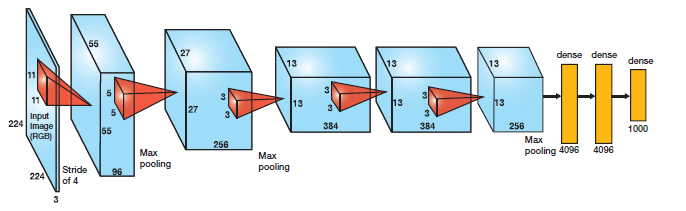AlexNet Model Architecture

The AlexNet contains 5 convolutional layers followed by 3 fully connected layer and takes in an input of shape 227x227x3. Besides the architecture, this model differs from the LeNet as below:

1. Uses max pooling instead of average pooling
2. Uses padding in some layers
3. Uses ReLU as its non-linearity function
4. Uses dropout after each fully connected layer

### VGG16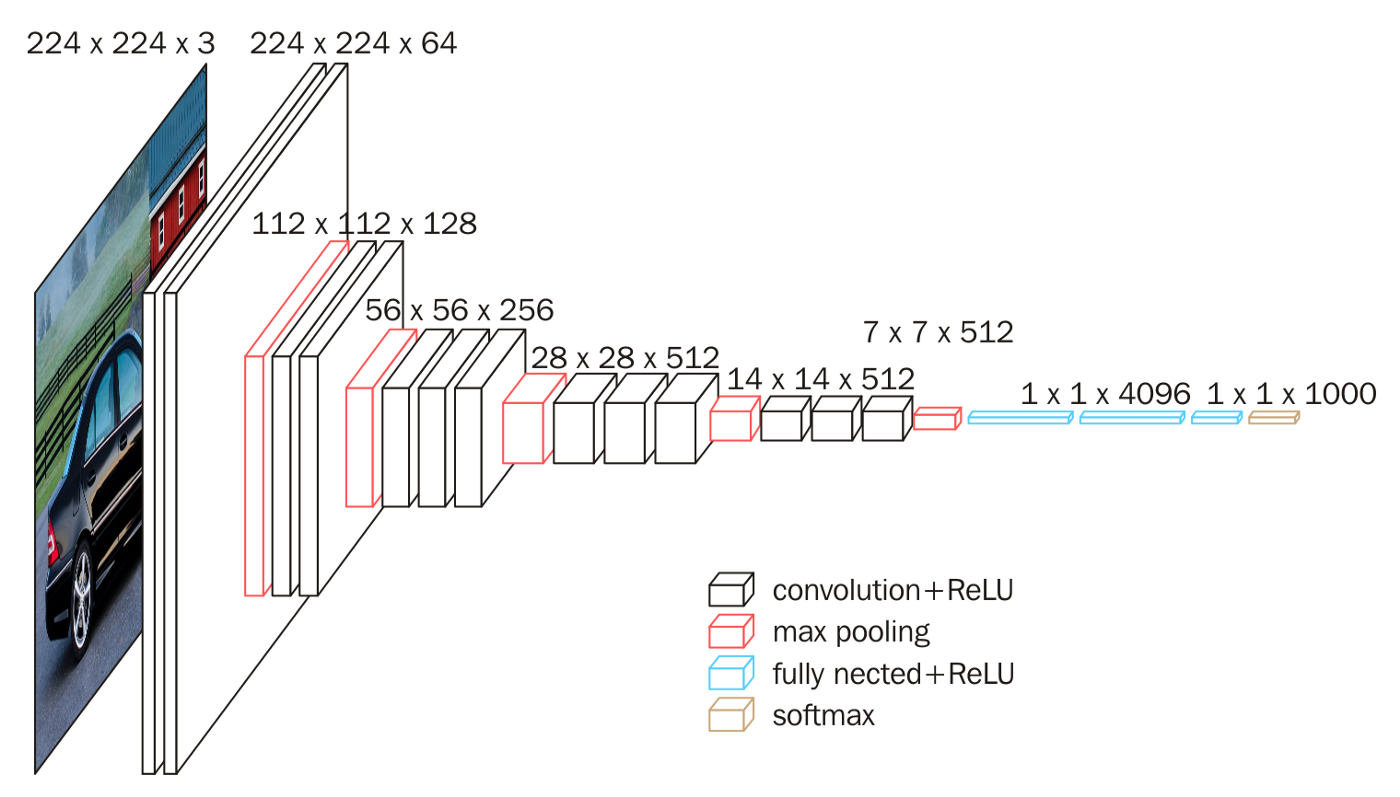The VGG16 differs from the AlexNet in that it uses 3x3 convolution for all convolutional layers and also uses a max pooling of 2x2. After the convolutional layers, it is followed by 3 fully connected layers. The number 16 in VGG16 signifies that there are 16 layers that has weights.

We can see that as we go deeper, the height and width decreases by a factor of 2 while the number of channels doubles.

### ResNets

From our intuition, a neural network accuracy should increase as we go deeper. However, a problem with very deep neural network is vanishing gradient. The concept of vanishing gradient is that during back propagation, as we move backward, the gradients tend to become smaller. This will make the earlier layers learn slowly compared to later layers and will result in a decreasing accuracy. ResNet solves the problem of vanishing gradient for us, but before diving in to the residual network, we first need to understand what a residual block does.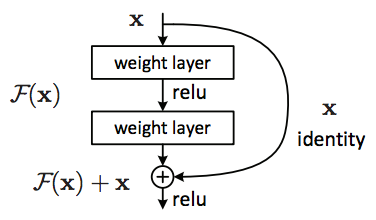A residual block differs from a normal neural network in the new connection that is added which some people also call it a skip connection. This is because the information from the previous layer besides going in the normal direction is also passed directly to the next node as well.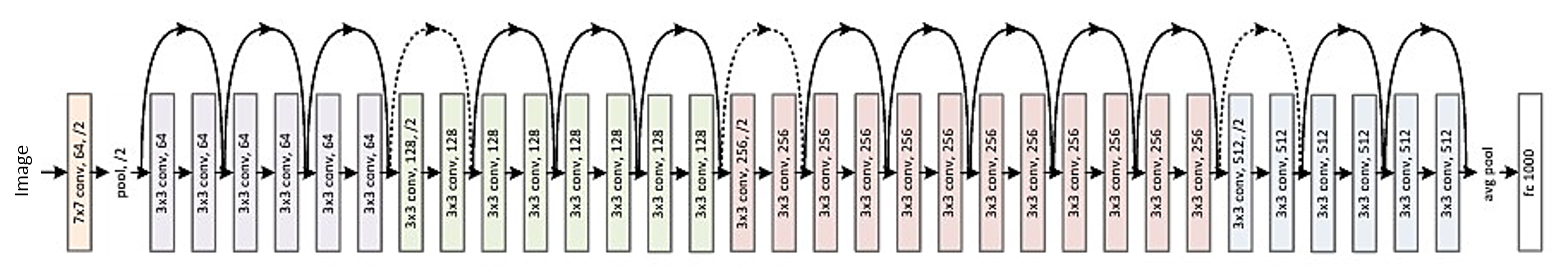ResNet Model Architecture

ResNet is just a neural network made up of one or more residual blocks.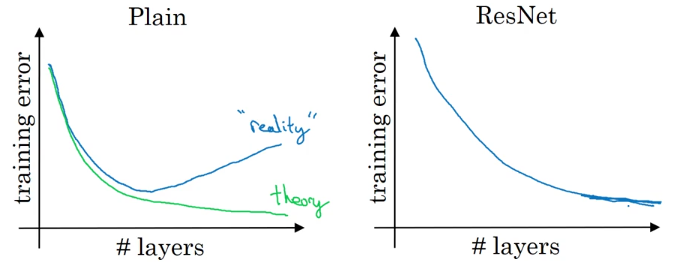We can see that, in ResNet, as we increase the number of layers, the training error decreases which is not always true in a plain neural network.

### Inception Network

The motivation behind an inception network is instead of choosing the size of convolution layer, or whether we want a pooling or convolutional layer, we try them all. After trying all our options, we concatenate the output together. This enforces us to use padding in order to make the width and height of each block to have to same dimension so that we can concatenate the outputs.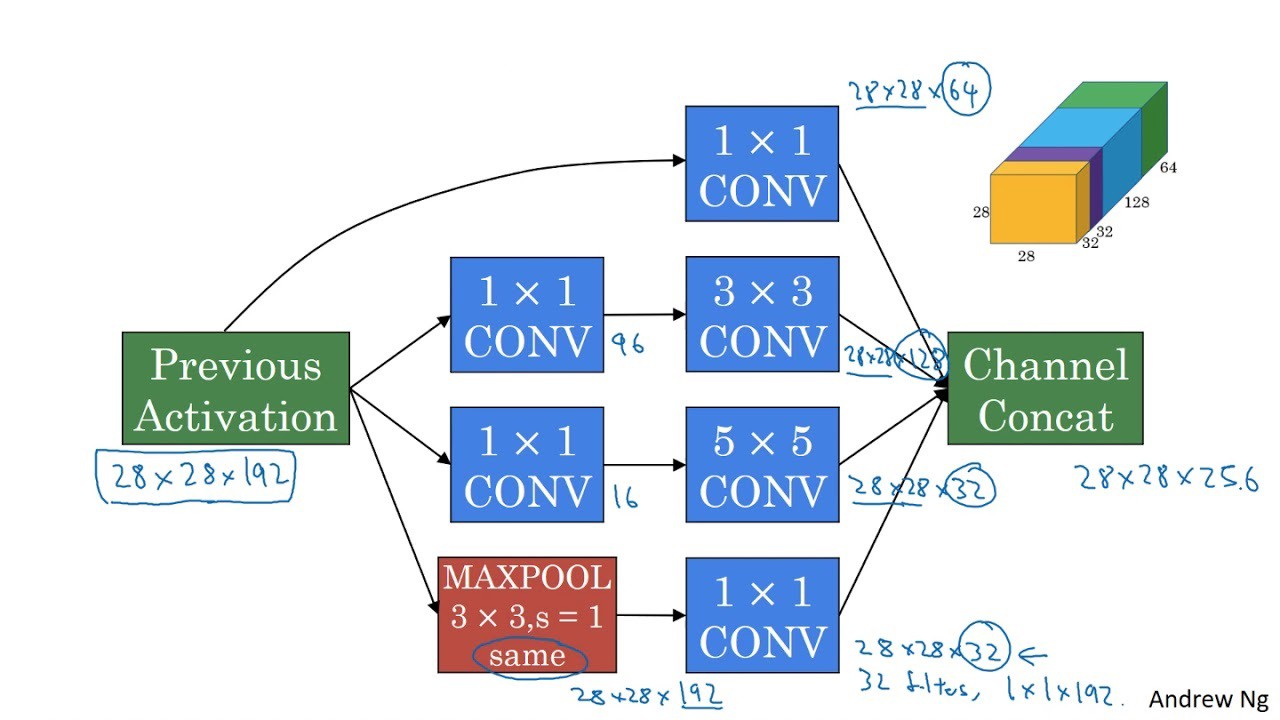A problem with the inception network is the number of calculations we have to make this is why we introduce the concept of a 1x1 convolution. A 1x1 convolution allows us to reduce the dimension which will reduce the computation we have to make.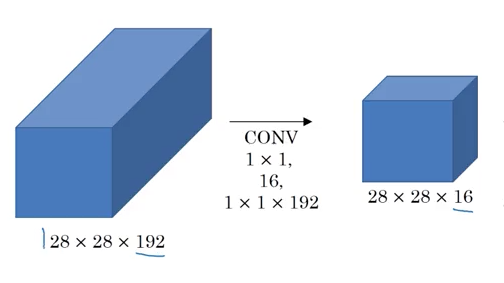AlexNet Model Architecture

By combining many inception units, we have the inception network as below.